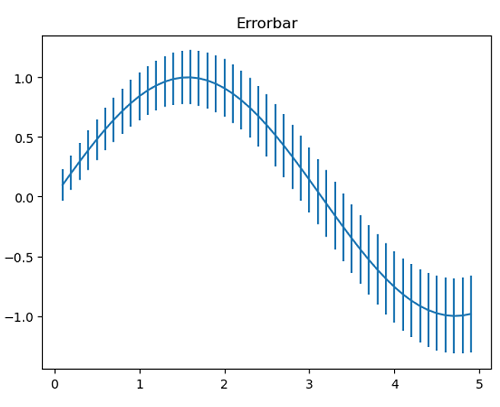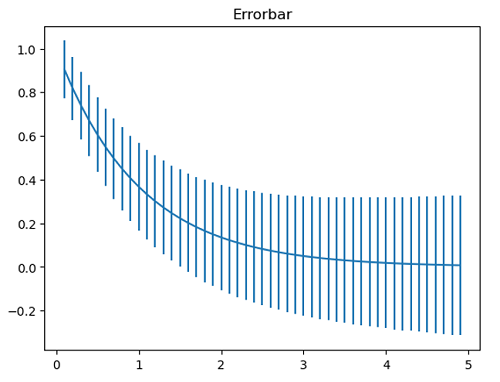# Python | Error-Bar in Plotting

Python | Error-Bar in Plotting: In this article, we are going to learn how to add error-bar in plotting in Python?
Submitted by Anuj Singh, on July 16, 2020

It is one of the most important aspects of plotting. Because of the huge application in experimental Data Visualization, matplotlib has provided a function matplotlib.pyplot.errorbar() for our desired operations.## Python code to demonstrate example of error-bar in plotting

```import numpy as np
import matplotlib.pyplot as plt

x = np.arange(0.1, 5, 0.1)
y = np.sin(x)
yerr = 0.1 + 0.1 * np.sqrt(x)

plt.figure()
plt.errorbar(x, y, yerr=yerr)
plt.title('Errorbar')
plt.show()

y = np.exp(-x)
yerr = 0.1 + 0.1 * np.sqrt(x)

plt.figure()
plt.errorbar(x, y, yerr=yerr)
plt.title('Errorbar')
plt.show()
```

Output:

```Output is as figure
```

Languages: » C » C++ » C++ STL » Java » Data Structure » C#.Net » Android » Kotlin » SQL
Web Technologies: » PHP » Python » JavaScript » CSS » Ajax » Node.js » Web programming/HTML
Solved programs: » C » C++ » DS » Java » C#
Aptitude que. & ans.: » C » C++ » Java » DBMS
Interview que. & ans.: » C » Embedded C » Java » SEO » HR
CS Subjects: » CS Basics » O.S. » Networks » DBMS » Embedded Systems » Cloud Computing
» Machine learning » CS Organizations » Linux » DOS
More: » Articles » Puzzles » News/Updates